SVG Tiny 1.2 - 20051207

# 7 Coordinate Systems, Transformations and Units

## 7.1 Introduction

For all media, the SVG canvas describes "the space where the SVG content is rendered." The canvas is infinite for each dimension of the space, but rendering occurs relative to a finite rectangular region of the canvas. This finite rectangular region is called the SVG viewport. For visual media [ CSS2-VISUAL], the SVG viewport is the viewing area where the user sees the SVG content.

The size of the SVG viewport (i.e., its width and height) is determined by a negotiation process (see Establishing the size of the initial viewport) between the SVG document fragment and its parent (real or implicit). Once that negotiation process is completed, the SVG user agent is provided the following information:

• a number (usually an integer) that represents the width in "pixels" of the viewport
• a number (usually an integer) that represents the height in "pixels" of the viewport
• (highly desirable but not required) a real number value that indicates the size in real world units, such as millimeters, of a "pixel" (i.e., a px unit as defined in [ CSS2 lengths])

Using the above information, the SVG user agent determines the viewport, an initial viewport coordinate system and an initial user coordinate system such that the two coordinates systems are identical. Both coordinates systems are established such that the origin matches the origin of the viewport (for the rootmost viewport, the viewport origin is at the top/left corner), and one unit in the initial coordinate system equals one "pixel" in the viewport. (See Initial coordinate system.) The viewport coordinate system is also called viewport space and the user coordinate system is also called user space.

Lengths in SVG are specified as values in user space (e.g. "15") and in a couple of special cases with an additional absolute unit measure (e.g. "15mm"). See Units for details.

A new user space (i.e., a new current coordinate system) can be established at any place within an SVG document fragment by specifying transformations in the form of transformation matrices or simple transformation operations such as rotation, skewing, scaling and translation. Establishing new user spaces via coordinate system transformations are fundamental operations to 2D graphics and represent the usual method of controlling the size, position, rotation and skew of graphic objects.

New viewports also can be established. By establishing a new viewport, you can provide a new reference rectangle for "fitting" a graphic into a particular rectangular area. ("Fit" means that a given graphic is transformed in such a way that its bounding box in user space aligns exactly with the edges of a given viewport.)

## 7.2 The initial viewport

The SVG user agent negotiates with its parent user agent to determine the viewport into which the SVG user agent can render the document. In some circumstances, SVG content will be embedded (by reference or inline) within a containing document. This containing document might include attributes, properties and/or other parameters (explicit or implicit) which specify or provide hints about the dimensions of the viewport for the SVG content. SVG content itself optionally can provide information about the appropriate viewport region for the content via the width and height XML attributes on the 'svg' element. The negotiation process uses any information provided by the containing document and the SVG content itself to choose the viewport location and size.

The width attribute on the 'svg' element establishes the viewport's width, unless all of the following conditions are met:

• the SVG content is a separately stored resource that is embedded by reference (such as the 'object' element in [XHTML]), or the SVG content is embedded inline within a parent document;
• the parent document is styled using CSS [CSS2] or XSL [XSL];
• CSS-compatible width properties [see CSS2-VISUDET] or corresponding XSL properties are specified on the referencing element (or rootmost svg element for inline SVG content).

Under these conditions, the 'svg' element must be treated as a replaced element in a CSS context, so the positioning properties establish the viewport's width.

Similarly, if CSS-compatible height properties or corresponding XSL properties on the referencing element (or rootmost svg element for inline SVG content), then these positioning properties establish the viewport's height; otherwise, the height attribute on the 'svg' element establishes the viewport's height.

If the width or height attributes on the 'svg' element are in user units (i.e., no unit identifier has been provided), then the value is assumed to be equivalent to the same number of "px" units (see Units).

In the following example, an SVG graphic is embedded inline within a parent XML document which is formatted using CSS layout rules. The width="100px" and height="200px" attributes determine the size of the initial viewport:

Example: 07_01.xml
```<?xml version="1.0"?>
<parent xmlns="http://www.example.org">
<!-- SVG graphic -->
<svg xmlns='http://www.w3.org/2000/svg'
width="100px" height="200px" version="1.2" baseProfile="tiny">
<path d="M100,100 Q200,400,300,100"/>
<!-- rest of SVG graphic would go here -->
</svg>
</parent>
```

## 7.3 The initial coordinate system

For the 'svg' element, the SVG user agent determines an initial viewport coordinate system and an initial user coordinate system such that the two coordinates systems are identical. The origin of both coordinate systems is at the origin of the viewport, and one unit in the initial coordinate system equals one "pixel" (i.e., a px unit as defined in [ CSS2 lengths]) in the viewport. In most cases, such as stand-alone SVG documents or SVG document fragments embedded (by reference or inline) within XML parent documents where the parent's layout is determined by CSS [CSS2] or XSL [XSL], the initial viewport coordinate system (and therefore the initial user coordinate system) has its origin at the top/left of the viewport, with the positive x-axis pointing towards the right, the positive y-axis pointing down, and text rendered with an "upright" orientation, which means glyphs are oriented such that Roman characters and full-size ideographic characters for Asian scripts have the top edge of the corresponding glyphs oriented upwards and the right edge of the corresponding glyphs oriented to the right.

If the SVG implementation is part of a user agent which supports styling XML documents using CSS2-compatible px units, then the SVG user agent should get its initial value for the size of a px unit in real world units to match the value used for other XML styling operations; otherwise, if the user agent can determine the size of a px unit from its environment, it should use that value; otherwise, it should choose an appropriate size for one px unit. In all cases, the size of a px must be in conformance with the rules described in [ CSS2 lengths].

Example 07_02 below shows that the initial coordinate system has the origin at the top/left with the x-axis pointing to the right and the y-axis pointing down. The initial user coordinate system has one user unit equal to the parent (implicit or explicit) user agent's "pixel".

Example: 07_02.svg
```﻿<?xml version="1.0"?>
<svg width="300px" height="100px" version="1.2" baseProfile="tiny"
xmlns="http://www.w3.org/2000/svg">
<desc>Example InitialCoords - SVG's initial coordinate system</desc>
<g fill="none" stroke="black" stroke-width="3" >
<line x1="0" y1="1.5" x2="300" y2="1.5" />
<line x1="1.5" y1="0" x2="1.5" y2="100" />
</g>
<g fill="red" stroke="none" >
<rect x="0" y="0" width="3" height="3" />
<rect x="297" y="0" width="3" height="3" />
<rect x="0" y="97" width="3" height="3" />
</g>
<g font-size="14" font-family="Verdana" >
<text x="10" y="20">(0,0)</text>
<text x="240" y="20">(300,0)</text>
<text x="10" y="90">(0,100)</text>
</g>
</svg>
```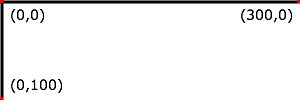## 7.4 Coordinate system transformations

A new user space (i.e., a new current coordinate system) can be established by specifying transformations in the form of a transform attribute on a container element or graphics element or a viewBox attribute on the 'svg' element. The transform and viewBox attributes transform user space coordinates and lengths on sibling attributes on the given element (see effect of the transform attribute on sibling attributes and effect of the viewBox attribute on sibling attributes) and all of its descendants. Transformations can be nested, in which case the effect of the transformations are cumulative.

Example 07_03 below shows a document without transformations. The text string is specified in the initial coordinate system.

Example: 07_03.svg
```﻿<?xml version="1.0"?>
<svg width="400px" height="150px" version="1.2" baseProfile="tiny"
xmlns="http://www.w3.org/2000/svg">
<desc>Example OrigCoordSys - Simple transformations: original picture</desc>
<g fill="none" stroke="black" stroke-width="3" >
<!-- Draw the axes of the original coordinate system -->
<line x1="0" y1="1.5" x2="400" y2="1.5" />
<line x1="1.5" y1="0" x2="1.5" y2="150" />
</g>
<g>
<text x="30" y="30" font-size="20" font-family="Verdana" >
ABC (orig coord system)
</text>
</g>
</svg>
```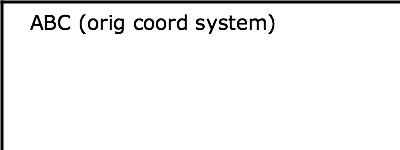Example 07_04 establishes a new user coordinate system by specifying transform="translate(50,50)" on the third 'g' element below. The new user coordinate system has its origin at location (50,50) in the original coordinate system. The result of this transformation is that the coordinate (30,30) in the new user coordinate system gets mapped to coordinate (80,80) in the original coordinate system (i.e., the coordinates have been translated by 50 units in X and 50 units in Y).

Example: 07_04.svg
```﻿<?xml version="1.0"?>
<svg width="400px" height="150px" version="1.2" baseProfile="tiny"
xmlns="http://www.w3.org/2000/svg">
<desc>Example NewCoordSys - New user coordinate system</desc>
<g fill="none" stroke="black" stroke-width="3" >
<!-- Draw the axes of the original coordinate system -->
<line x1="0" y1="1.5" x2="400" y2="1.5" />
<line x1="1.5" y1="0" x2="1.5" y2="150" />
</g>
<g>
<text x="30" y="30" font-size="20" font-family="Verdana" >
ABC (orig coord system)
</text>
</g>
<!-- Establish a new coordinate system, which is
shifted (i.e., translated) from the initial coordinate
system by 50 user units along each axis. -->
<g transform="translate(50,50)">
<g fill="none" stroke="red" stroke-width="3" >
<!-- Draw lines of length 50 user units along
the axes of the new coordinate system -->
<line x1="0" y1="0" x2="50" y2="0" stroke="red" />
<line x1="0" y1="0" x2="0" y2="50" />
</g>
<text x="30" y="30" font-size="20" font-family="Verdana" >
ABC (translated coord system)
</text>
</g>
</svg>
```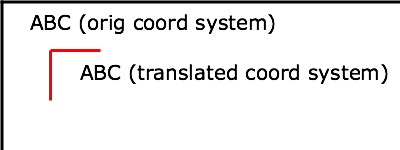Example 07_05 illustrates simple rotate and scale transformations. The example defines two new coordinate systems:

• one which is the result of a translation by 50 units in X and 30 units in Y, followed by a rotation of 30 degrees
• another which is the result of a translation by 200 units in X and 40 units in Y, followed by a scale transformation of 1.5.
Example: 07_05.svg
```﻿<?xml version="1.0"?>
<svg width="400px" height="120px" version="1.2" baseProfile="tiny"
xmlns="http://www.w3.org/2000/svg">
<desc>Example RotateScale - Rotate and scale transforms</desc>
<g fill="none" stroke="black" stroke-width="3" >
<!-- Draw the axes of the original coordinate system -->
<line x1="0" y1="1.5" x2="400" y2="1.5" />
<line x1="1.5" y1="0" x2="1.5" y2="120" />
</g>
<!-- Establish a new coordinate system whose origin is at (50,30)
in the initial coord. system and which is rotated by 30 degrees. -->
<g transform="translate(50,30)">
<g transform="rotate(30)">
<g fill="none" stroke="red" stroke-width="3" >
<line x1="0" y1="0" x2="50" y2="0" />
<line x1="0" y1="0" x2="0" y2="50" />
</g>
<text x="0" y="0" font-size="20" font-family="Verdana" fill="blue" >
ABC (rotate)
</text>
</g>
</g>
<!-- Establish a new coordinate system whose origin is at (200,40)
in the initial coord. system and which is scaled by 1.5. -->
<g transform="translate(200,40)">
<g transform="scale(1.5)">
<g fill="none" stroke="red" stroke-width="3" >
<line x1="0" y1="0" x2="50" y2="0" />
<line x1="0" y1="0" x2="0" y2="50" />
</g>
<text x="0" y="0" font-size="20" font-family="Verdana" fill="blue" >
ABC (scale)
</text>
</g>
</g>
</svg>
```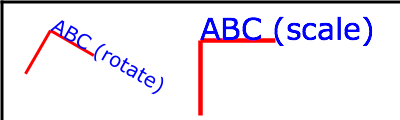Example 07_06 defines two coordinate systems which are skewed relative to the origin coordinate system.

Example: 07_06.svg
```﻿<?xml version="1.0"?>
<svg width="400px" height="120px" version="1.2" baseProfile="tiny"
xmlns="http://www.w3.org/2000/svg">
<desc>Example Skew - Show effects of skewX and skewY</desc>
<g fill="none" stroke="black" stroke-width="3" >
<!-- Draw the axes of the original coordinate system -->
<line x1="0" y1="1.5" x2="400" y2="1.5" />
<line x1="1.5" y1="0" x2="1.5" y2="120" />
</g>
<!-- Establish a new coordinate system whose origin is at (30,30)
in the initial coord. system and which is skewed in X by 30 degrees. -->
<g transform="translate(30,30)">
<g transform="skewX(30)">
<g fill="none" stroke="red" stroke-width="3" >
<line x1="0" y1="0" x2="50" y2="0" />
<line x1="0" y1="0" x2="0" y2="50" />
</g>
<text x="0" y="0" font-size="20" font-family="Verdana" fill="blue" >
ABC (skewX)
</text>
</g>
</g>
<!-- Establish a new coordinate system whose origin is at (200,30)
in the initial coord. system and which is skewed in Y by 30 degrees. -->
<g transform="translate(200,30)">
<g transform="skewY(30)">
<g fill="none" stroke="red" stroke-width="3" >
<line x1="0" y1="0" x2="50" y2="0" />
<line x1="0" y1="0" x2="0" y2="50" />
</g>
<text x="0" y="0" font-size="20" font-family="Verdana" fill="blue" >
ABC (skewY)
</text>
</g>
</g>
</svg>
```Mathematically, all transformations can be represented as 3x3 transformation matrices of the following form: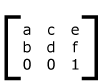Since only six values are used in the above 3x3 matrix, a transformation matrix is also expressed as a vector: [a b c d e f].

Transformations map coordinates and lengths from a new coordinate system into a previous coordinate system: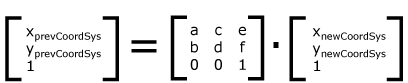Simple transformations are represented in matrix form as follows:

• Translation is equivalent to the matrix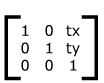or [1 0 0 1 tx ty], where tx and ty are the distances to translate coordinates in X and Y, respectively.

• Scaling is equivalent to the matrix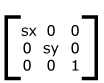or [sx 0 0 sy 0 0]. One unit in the X and Y directions in the new coordinate system equals sx and sy units in the previous coordinate system, respectively.

• Rotation about the origin is equivalent to the matrix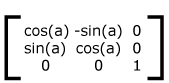or [cos(a) sin(a) -sin(a) cos(a) 0 0], which has the effect of rotating the coordinate system axes by angle a.

• A skew transformation along the x-axis is equivalent to the matrix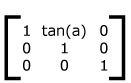or [1 0 tan(a) 1 0 0], which has the effect of skewing X coordinates by angle a.

• A skew transformation along the y-axis is equivalent to the matrixor [1 tan(a) 0 1 0 0], which has the effect of skewing Y coordinates by angle a.

## 7.5 Nested transformations

Transformations can be nested to any level. The effect of nested transformations is to post-multiply (i.e., concatenate) the subsequent transformation matrices onto previously defined transformations:For each given element, the accumulation of all transformations that have been defined on the given element and all of its ancestors up to and including the element that established the current viewport (usually, the 'svg' element which is the most immediate ancestor to the given element) is called the current transformation matrix or CTM. The CTM thus represents the mapping of current user coordinates to viewport coordinates: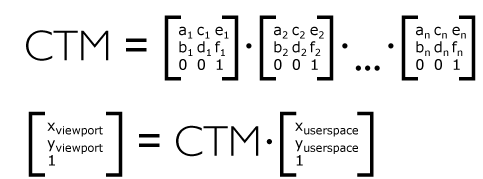Example 07_07 illustrates nested transformations.

Example: 07_07.svg
```﻿<?xml version="1.0"?>
<svg width="400px" height="150px" version="1.2" baseProfile="tiny"
xmlns="http://www.w3.org/2000/svg">
<desc>Example Nested - Nested transformations</desc>
<g fill="none" stroke="black" stroke-width="3" >
<!-- Draw the axes of the original coordinate system -->
<line x1="0" y1="1.5" x2="400" y2="1.5" />
<line x1="1.5" y1="0" x2="1.5" y2="150" />
</g>
<!-- First, a translate -->
<g transform="translate(50,90)">
<g fill="none" stroke="red" stroke-width="3" >
<line x1="0" y1="0" x2="50" y2="0" />
<line x1="0" y1="0" x2="0" y2="50" />
</g>
<text x="0" y="0" font-size="16" font-family="Verdana" >
....Translate(1)
</text>
<!-- Second, a rotate -->
<g transform="rotate(-45)">
<g fill="none" stroke="green" stroke-width="3" >
<line x1="0" y1="0" x2="50" y2="0" />
<line x1="0" y1="0" x2="0" y2="50" />
</g>
<text x="0" y="0" font-size="16" font-family="Verdana" >
....Rotate(2)
</text>
<!-- Third, another translate -->
<g transform="translate(130,160)">
<g fill="none" stroke="blue" stroke-width="3" >
<line x1="0" y1="0" x2="50" y2="0" />
<line x1="0" y1="0" x2="0" y2="50" />
</g>
<text x="0" y="0" font-size="16" font-family="Verdana" >
....Translate(3)
</text>
</g>
</g>
</g>
</svg>
```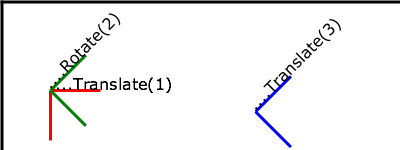In the example above, the CTM within the third nested transformation (i.e., the transform="translate(130,160)") consists of the concatenation of the three transformations, as follows: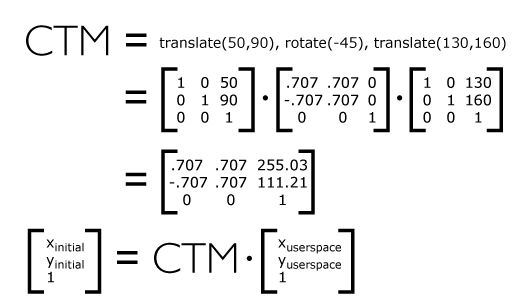## 7.6 The transform attribute

transform = <transform-list> | ref(svg [, <x>, <y>])

For the transform attribute:

Animatable: yes.

See the 'animateTransform' element for information on animating transformations.

The <transform-list> attribute type is defined below. The 'ref( )' attribute type is defined in the Constrained Transformations section.

### 7.6.1 The TransformList value

A <transform-list> is defined as a list of transform definitions, which are applied in the order provided. The individual transform definitions are separated by whitespace and/or a comma. The available types of transform definitions include:

• matrix(<a> <b> <c> <d> <e> <f>), which specifies a transformation in the form of a transformation matrix of six values. matrix(a,b,c,d,e,f) is equivalent to applying the transformation matrix [a b c d e f].

• translate(<tx> [<ty>]), which specifies a translation by tx and ty. If <ty> is not provided, it is assumed to be zero.

• scale(<sx> [<sy>]), which specifies a scale operation by sx and sy. If <sy> is not provided, it is assumed to be equal to <sx>.

• rotate(<rotate-angle> [<cx> <cy>]), which specifies a rotation by <rotate-angle> degrees about a given point.

If optional parameters <cx> and <cy> are not supplied, the rotate is about the origin of the current user coordinate system. The operation corresponds to the matrix [cos(a) sin(a) -sin(a) cos(a) 0 0].

If optional parameters <cx> and <cy> are supplied, the rotate is about the point (<cx>, <cy>). The operation represents the equivalent of the following specification: translate(<cx>, <cy>) rotate(<rotate-angle>) translate(-<cx>, -<cy>).

• skewX(<skew-angle>), which specifies a skew transformation along the x-axis.

• skewY(<skew-angle>), which specifies a skew transformation along the y-axis.

All numeric values are real <number>s.

A matrix with all values = 0 (`matrix(0,0,0,0,0,0)`) disables the rendering of the element, it does not create an error.

If a list of transforms is provided, then the net effect is as if each transform had been specified separately in the order provided. For example,

Example: 07_08.svg
```<g transform="translate(-10,-20) scale(2) rotate(45) translate(5,10)">
<!-- graphics elements go here -->
</g>
```

is functionally equivalent to:

Example: 07_09.svg
```<g transform="translate(-10,-20)">
<g transform="scale(2)">
<g transform="rotate(45)">
<g transform="translate(5,10)">
<!-- graphics elements go here -->
</g>
</g>
</g>
</g>
```

The transform attribute is applied to an element before processing any other coordinate or length values supplied for that element. In the element

Example: 07_10.svg
`<rect x="10" y="10" width="20" height="20" transform="scale(2)"/>    `

the x, y, width and height values are processed after the current coordinate system has been scaled uniformly by a factor of 2 by the transform attribute. Attributes x, y, width and height (and any other attributes or properties) are treated as values in the new user coordinate system, not the previous user coordinate system. Thus, the above 'rect' element is functionally equivalent to:

Example: 07_11.svg
```<g transform="scale(2)">
<rect x="10" y="10" width="20" height="20"/>
</g>
```

The following is the Backus-Naur Form (BNF) for values for <transform-list>. The following notation is used:

• *: 0 or more
• +: 1 or more
• ?: 0 or 1
• (): grouping
• |: separates alternatives
• double quotes surround literals
```transform-list:
wsp* transforms? wsp*
transforms:
transform
| transform comma-wsp+ transforms
transform:
matrix
| translate
| scale
| rotate
| skewX
| skewY
matrix:
"matrix" wsp* "(" wsp*
number comma-wsp
number comma-wsp
number comma-wsp
number comma-wsp
number comma-wsp
number wsp* ")"
translate:
"translate" wsp* "(" wsp* number ( comma-wsp number )? wsp* ")"
scale:
"scale" wsp* "(" wsp* number ( comma-wsp number )? wsp* ")"
rotate:
"rotate" wsp* "(" wsp* number ( comma-wsp number comma-wsp number )? wsp* ")"
skewX:
"skewX" wsp* "(" wsp* number wsp* ")"
skewY:
"skewY" wsp* "(" wsp* number wsp* ")"
number:
sign? integer-constant
| sign? floating-point-constant
comma-wsp:
(wsp+ comma? wsp*) | (comma wsp*)
comma:
","
integer-constant:
digit-sequence
floating-point-constant:
fractional-constant exponent?
| digit-sequence exponent
fractional-constant:
digit-sequence? "." digit-sequence
| digit-sequence "."
exponent:
( "e" | "E" ) sign? digit-sequence
sign:
"+" | "-"
digit-sequence:
digit
| digit digit-sequence
digit:
"0" | "1" | "2" | "3" | "4" | "5" | "6" | "7" | "8" | "9"
wsp:
(#x20 | #x9 | #xD | #xA)
```

## 7.7 Constrained Transformations

SVG 1.2 extends the coordinate system transformations allowed on groups and graphical elements to provide a method by which graphical objects can remain fixed in the viewport without being scaled or rotated. Use cases include thin lines that do not become fatter on zooming in, map symbols or icons of a constant size, and so forth.

The following summarizes the different transforms that are applied to a graphical object as it is rendered.

### 7.7.1 The User Transform

The User Transform is the transformation that the user agent positioning controls apply to the viewport coordinate system. This transform can be considered to be applied to a group that surrounds the svg element of the document.

The user agent positioning controls consist of a translation (commonly referred to as the "pan"), a scale (commonly referred to as the "zoom") and a rotate.

```US = User Scale (currentScale on SVGSVGElement)
UP = User Pan (currentTranslate on SVGSVGElement)
UR = User Rotate (currentRotate on SVGSVGElement)```

The User Transform is the product of these component transformations.

```U = User Transform
= UP.US.UR```

### 7.7.2 ViewBox to Viewport transformation

SVG elements, such as the rootmost svg element, create their own viewport. The viewBox to viewport transformation is the transformation on an svg element that adjusts the coordinate system to take the viewBox and preserveAspectRatio attributes into account.

We use the following notation for a viewBox to viewport transformation:

`VB(svgId)`

The 'svgId' parameter is the value of the id or xml:id attribute on a given svg element.

### 7.7.3 Element Transform Stack

All elements in an SVG document have a transform stack. This is the list of transforms that manipulate the coordinate system between the element and its nearest ancestor svg element, i.e. in this specification, the root element.

We use the following notation for the Element Transform stack on a given element:

`TS(id)`

The 'id' parameter is the value of the id or xml:id attribute on a given element.

Similarly, we use the following notation for the the transform defined by the transform attribute on the given element with identifier 'elt'.

`Txf(id)`

With the above definition, the transformation TS of an element is equal to the product of all the transformations Txf from that element to its neareast ancestor svg.

`TS(id) = Txf(id.nearestViewportElement).[...].Txf(id.parentElement).Txf(id)`
Example: Element transform stack
```<svg id="root" version="1.2" baseProfile="tiny">
<g id="g" transform="scale(2)">
<rect id="r" transform="scale(4)"/>
<g id="g2">
<rect id="r2" transform="scale(0.5)" />
</g>
</g>
</svg>
```

In this example, the transforms are:

```TS(g) = scale(2)
TS(r) = TS(g) . scale(4) = scale(8)
TS(g2) = TS(g) . I = scale(4)  (I is the identity matrix)
TS(r2) = TS(g) . scale(0.5) = scale(1)

```

### 7.7.4 The Current Transform Matrix

Each element in the rendering tree has the concept of a Current Transform Matrix, or CTM. This is the product of all coordinate system transformations that apply to an element, effectively mapping the element into a coordinate system that is then transformed into device units by the user agent.

Consider the following example, with a rectangle having a set of ancestor g elements with ids "g-0" to "g-n".

Example: Current transform matrix
```<svg id="root" version="1.2" baseProfile="tiny">
...
<g id="g-n">
...
<g id="g-2">
...
<g id="g-1">
...
<g id="g-0">
...
<rect id="elt" .../>
</g>
</g>
</g>
</g>
</svg>
```

With the above definitions for U, VB, and TS, the CTM for the rectangle with id "elt" is:

```CTM(elt) = U.VB(root).TS(elt)
= U.VB(root). Txf(g-n).[...].Txf(g-0).Txf(elt)
```
Example: n=2
```<svg id="root" version="1.2" baseProfile="tiny">
...
<g id="g-1">
...
<g id="g-0">
...
<rect id="elt" .../>
</g>
</g>
</svg>
```

This produces the following transformations:

```CTM(elt) = U.VB(root).Txf(g-1).Txf(g-0).Txf(elt)
```

Note the important relationship between an element's CTM and its parent CTM, for elements which do not define a viewport:

`CTM(elt) = CTM(elt.parentElement).Txf(elt)`

### 7.7.5 The ref() transform value

By using the "ref()" attribute value on the transform attribute it is possible to provide simple constrained transformations.

The 'ref(svg, x, y)' transform evaluates to the inverse of the element's parent's CTM multiplied by the svg element's CTM but exclusive of the svg element's zoom/pan/rotate user transform, if any.

The x and y parameters are optional. If they are specified an additional translation is appended to the transform so that (0, 0) in the element's user space maps to (x, y) in the svg element's user space. If no x and y parameters are specified, no additional translation is applied.

Using the definitions provided above:

```Inverse of the parent's CTM: inv(CTM(elt.parentElement))

The svg element's user transform, exclusive of zoom,
pan and rotate transforms:
CTM(svg.parentElement).VB(svg)

CTM(svg.parentElement) evaluates to Identity since there
is no svg.parentElement element.```

In addition, the T(x, y) translation is such that:

`CTM(elt).(0, 0) = CTM(svg).(x, y)`

So the transform evaluates to:

`Txf(elt) = inv(CTM(elt.parentElement)).CTM(svg.parentElement).VB(svg).T(x, y)`

The element's CTM is:

```CTM(elt) = CTM(elt.parentElement).Txf(elt)
= CTM(svg.parentElement).VB(svg).T(x,y)```
Example: ref() transform

A small rectangle initially marks the middle of a line. The user agent viewport is a square with sides of 200 units.
```<svg id="root" viewBox="0 0 100 100" version="1.2" baseProfile="tiny"><line x1="0" x2="100" y1="0" y2="100"/><rect id="r" transform="ref(svg)"x="45" y="45" width="10" height="10"/></svg>
```

In this case:

```Txf(r) = inv(CTM(r.parent)).CTM(root.parentElement).VB(root).T(x, y)CTM(root.parentElement) evaluates to Identity.T(x, y) evaluates to Identity because (x, y) is not specifiedCTM(r) = CTM(r.parent).Txf(r)= CTM(r.parent).inv(CTM(r.parent)).VB(root)= VB(root)= scale(2)
```

Consequently, regardless of the user transform (currentTranslate, currentScale, currentRotate) the rectangle's coordinates in viewport space will always be: (45, 45, 10, 10)*scale(2) = (90, 90, 20, 20). Initially, the line is from (0, 0) to (200, 200) in the viewport coordinate system. If we apply a user agent zoom of 3 (currentScale = 3), the rectangle is still (90, 90, 20, 20) but the line is (0, 0, 600, 600) and the marker no longer marks the middle of the line.

Example: ref() transform

A small rectangle always marks the middle of a line. Again, the user agent viewport is a square with sides of 200 units.
```<svg id="root" baseProfile="tiny" viewBox="0 0 100 100" version="1.2"> <line x1="0" x2="100" y1="0" y2="100"/><g id="g" transform="ref(svg, 50, 50)"><rect id="r" x="-5" y="-5" width="10" height="10"/></g></svg>
```

In this case:

```Txf(g) = inv(CTM(g.parent)).CTM(root.parentElement).VB(root).T(x,y)CTM(root.parentElement) evaluates to Identity.CTM(g) = CTM(g.parent).Txf(r)= CTM(g.parent).inv(CTM(g.parent)).VB(root).T(x,y)= VB(root).T(x,y)= scale(2).T(x,y)
```

Initially, (50, 50) in the svg user space is (100, 100) in viewport space. Therefore:

```CTM(g).[0, 0] = CTM(root).[50, 50]= scale(2).[50, 50]= [100, 100]andscale(2).T(x,y) = [100, 100]T(x,y) = translate(50, 50)
```

If the user agent pan was (50, 80) (modifying currentTranslate) then we now have (50, 50) in the svg element's user space located at (150, 180) in the viewport space. This produces:

```CTM(g).[0, 0] = CTM(root).[50, 50]= translate(50, 80).scale(2).[50, 50]= [150, 180]andscale(2).T(x,y) = [150, 180]T(x, y) = translate(75, 90)
```

Therefore, regardless of the user transform, the rectangle will always overlap the middle of the line. Note that the rectangle will not rotate with the line (e.g., if currentRotate is set) and it will not scale either.

## 7.8 The viewBox attribute

It is often desirable to specify that a given set of graphics stretch to fit a particular container element. The viewBox attribute provides this capability. All elements that establish a new viewport (see elements that establish viewports) have attribute viewBox.

Attribute definition:

viewBox = "<list> | 'none'
<list>
A list of four numbers <min-x>, <min-y>, <width> and <height>, separated by whitespace and/or a comma, which specify a rectangle in user space which should be mapped to the bounds of the viewport established by the given element, taking into account attribute preserveAspectRatio. If specified, an additional transformation is applied to all descendants of the given element to achieve the specified effect.
'none'
Specifying a value of 'none' indicates that no viewBox should be used. This is exactly the same as not setting the viewBox at all.

Animatable: yes.

A negative value for <width> or <height> is unsupported. A value of zero disables rendering of the element.

Example 07_12 illustrates the use of the viewBox attribute on the 'svg' element to specify that the SVG content should stretch to fit bounds of the viewport.

Example: 07_12.svg
```﻿<?xml version="1.0"?>
<svg width="300px" height="200px" version="1.2" baseProfile="tiny"
viewBox="0 0 1500 1000" preserveAspectRatio="none"
xmlns="http://www.w3.org/2000/svg">
<desc>Example ViewBox - uses the viewBox
attribute to automatically create an initial user coordinate
system which causes the graphic to scale to fit into the
viewport no matter what size the viewport is.</desc>
<!-- This rectangle goes from (0,0) to (1500,1000) in user space.
Because of the viewBox attribute above,
the rectangle will end up filling the entire area
reserved for the SVG content. -->
<rect x="0" y="0" width="1500" height="1000"
fill="yellow" stroke="blue" stroke-width="12"  />
<!-- A large, red triangle -->
<path fill="red"  d="M 750,100 L 250,900 L 1250,900 z"/>
<!-- A text string that spans most of the viewport -->
<text x="100" y="600" font-size="200" font-family="Verdana" >
Stretch to fit
</text>
</svg>
```
Rendered into viewport with width=300px, height=200px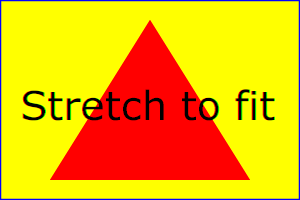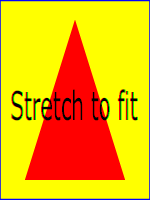The effect of the viewBox attribute is that the user agent automatically supplies the appropriate transformation matrix to map the specified rectangle in user space to the bounds of a designated region (often, the viewport). To achieve the effect of the example on the left, with viewport dimensions of 300 by 200 pixels, the user agent needs to automatically insert a transformation which scales both X and Y by 0.2. The effect is equivalent to having a viewport of size 300px by 200px and the following supplemental transformation in the document, as follows:

```<svg version="1.2" baseProfile="tiny" width="300px" height="200px"><g transform="scale(0.2)"><!-- Rest of document goes here --></g></svg>
```

To achieve the effect of the example on the right, with viewport dimensions of 150 by 200 pixels, the user agent needs to automatically insert a transformation which scales X by 0.1 and Y by 0.2. The effect is equivalent to having a viewport of size 150px by 200px and the following supplemental transformation in the document, as follows:

```<svg version="1.2" baseProfile="tiny" width="150px" height="200px"><g transform="scale(0.1 0.2)"><!-- Rest of document goes here --></g></svg>
```

(Note: in some cases the user agent will need to supply a translate transformation in addition to a scale transformation. For example, on an 'svg', a translate transformation will be needed if the viewBox attributes specifies values other than zero for <min-x> or <min-y>.)

Unlike the transform attribute (see effect of the transform on sibling attributes), the automatic transformation that is created due to a viewBox does not affect the x, y, width and height attributes on the element with the viewBox attribute. Thus, in the example above which shows an 'svg' element which has attributes width, height and viewBox, the width and height attributes represent values in the coordinate system that exists before the viewBox transformation is applied. On the other hand, like the transform attribute, it does establish a new coordinate system for all other attributes and for descendant elements.

## 7.9 The preserveAspectRatio attribute

In some cases, typically when using the viewBox attribute, it is desirable that the graphics stretch to fit non-uniformly to take up the entire viewport. In other cases, it is desirable that uniform scaling be used for the purposes of preserving the aspect ratio of the graphics.

Attribute preserveAspectRatio="[defer] <align> [<meet>]", which is available for all elements that establish a new viewport (see elements that establish viewports), indicates whether or not to force uniform scaling.

preserveAspectRatio only applies when a value has been provided for viewBox on the same element. Or, in some cases, if an implicit viewBox value can be established for the element (see each element description for details on this). If a viewBox value can not be determined then preserveAspectRatio is ignored.

If the value of preserveAspectRatio on an element that references data ('image', 'animation' and 'video') starts with 'defer' then the value of the preserveAspectRatio attribute on the referenced content if present should be used. If the referenced content lacks a value for preserveAspectRatio then the preserveAspectRatio attribute should be processed as normal (ignoring 'defer'). For preserveAspectRatio on all other elements the 'defer' portion of the attribute is ignored.

The <align> parameter indicates whether to force uniform scaling and, if so, the alignment method to use in case the aspect ratio of the viewBox doesn't match the aspect ratio of the viewport. The <align> parameter must be one of the following strings:

• none - Do not force uniform scaling. Scale the graphic content of the given element non-uniformly if necessary such that the element's bounding box exactly matches the viewport rectangle.

• xMinYMin - Force uniform scaling.

Align the <min-x> of the element's viewBox with the smallest X value of the viewport.

Align the <min-y> of the element's viewBox with the smallest Y value of the viewport.
• xMidYMin - Force uniform scaling.

Align the midpoint X value of the element's viewBox with the midpoint X value of the viewport.

Align the <min-y> of the element's viewBox with the smallest Y value of the viewport.
• xMaxYMin - Force uniform scaling.

Align the <min-x>+<width> of the element's viewBox with the maximum X value of the viewport.

Align the <min-y> of the element's viewBox with the smallest Y value of the viewport.
• xMinYMid - Force uniform scaling.

Align the <min-x> of the element's viewBox with the smallest X value of the viewport.

Align the midpoint Y value of the element's viewBox with the midpoint Y value of the viewport.
• xMidYMid (the default) - Force uniform scaling.

Align the midpoint X value of the element's viewBox with the midpoint X value of the viewport.

Align the midpoint Y value of the element's viewBox with the midpoint Y value of the viewport.
• xMaxYMid - Force uniform scaling.

Align the <min-x>+<width> of the element's viewBox with the maximum X value of the viewport.

Align the midpoint Y value of the element's viewBox with the midpoint Y value of the viewport.
• xMinYMax - Force uniform scaling.

Align the <min-x> of the element's viewBox with the smallest X value of the viewport.

Align the <min-y>+<height> of the element's viewBox with the maximum Y value of the viewport.
• xMidYMax - Force uniform scaling.

Align the midpoint X value of the element's viewBox with the midpoint X value of the viewport.

Align the <min-y>+<height> of the element's viewBox with the maximum Y value of the viewport.
• xMaxYMax - Force uniform scaling.

Align the <min-x>+<width> of the element's viewBox with the maximum X value of the viewport.

Align the <min-y>+<height> of the element's viewBox with the maximum Y value of the viewport.

The <meet> parameter is optional and is only available due to historical reasons. The <meet> is separated from the <align> value by one or more spaces and must equal the string meet. This is also the default value and therefore it is recommended that content do not specify this parameter since it adds no additional value.

meet indicates to scale the graphic such that:

• aspect ratio is preserved
• the entire viewBox is visible within the viewport
• the viewBox is scaled up as much as possible, while still meeting the other criteria

In this case, if the aspect ratio of the graphic does not match the viewport, some of the viewport will extend beyond the bounds of the viewBox (i.e., the area into which the viewBox will draw will be smaller than the viewport).

Example PreserveAspectRatio illustrates the various options on preserveAspectRatio. The example creates several new viewports by including 'animation' elements (see Establishing a new viewport).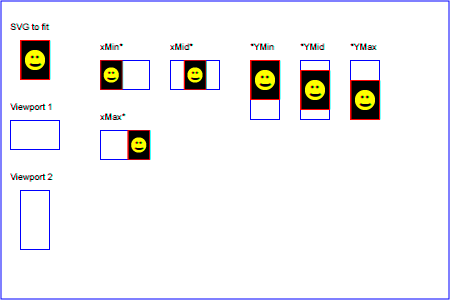For the preserveAspectRatio attribute:

Animatable: yes.

## 7.10 Establishing a new viewport

Some elements establish a new viewport. By establishing a new viewport, you implicitly establish a new viewport coordinate system and a new user coordinate system. Additionally, there is a new meaning for percentage units defined to be relative to the current viewport since a new viewport has been established (see Units)

'viewport-fill' and 'viewport-fill-opacity' properties can be applied on the new viewport.

The bounds of the new viewport are defined by the x, y, width and height attributes on the element establishing the new viewport, such as an 'animation' element. Both the new viewport coordinate system and the new user coordinate system have their origins at (x, y), where x and y represent the value of the corresponding attributes on the element establishing the viewport. The orientation of the new viewport coordinate system and the new user coordinate system correspond to the orientation of the current user coordinate system for the element establishing the viewport. A single unit in the new viewport coordinate system and the new user coordinate system are the same size as a single unit in the current user coordinate system for the element establishing the viewport.

For an extensive example of creating new viewports, see Example PreserveAspectRatio.

The following elements establish new viewports:

## 7.11 Units

Besides the exceptions listed below all coordinates and lengths in SVG must be specified in user units, witch means that unit identifiers are not allowed.

Two exceptions exist:

• unit identifiers are allowed on the 'width' and 'height' attributes of the 'svg' element.
• Object bounding box units are allowed on 'linearGradient' and 'radialGradient' elements.

A user unit is a value in the current user coordinate system. For example:

Example: 07_17.svg
`<text font-size="50">Text size is 50 user units</text>    `

For the svg element's 'width'and 'height' attributes a coordinate or length value can be expressed as a number following by a unit identifier (e.g., "25cm" or "100%"). The list of unit identifiers in SVG are the following CSS unit identifiers: in, cm, mm, pt, pc, px and percentages (%). The following describes how the various unit identifiers are processed:

• One px unit is defined to be equal to one user unit. Thus, a length of "5px" is the same as a length of "5".

• The other absolute unit identifiers from CSS (i.e., pt, pc, cm, mm, in) are all defined as an appropriate multiple of one px unit (which, according to the previous item, is defined to be equal to one user unit), based on what the SVG user agent determines is the size of a px unit (possibly passed from the parent processor or environment at initialization time). For example, suppose that the user agent can determine from its environment that "1px" corresponds to "0.2822222mm" (i.e., 90dpi). Then, for all processing:

• "1pt" equals "1.25px" (and therefore 1.25 user units)
• "1pc" equals "15px" (and therefore 15 user units)
• "1mm" would be "3.543307px" (3.543307 user units)
• "1cm" equals "35.43307px" (and therefore 35.43307 user units)
• "1in" equals "90px" (and therefore 90 user units)

Percentage values on 'width' and 'height' attributes mandates how much space the SVG viewport should take of the available initial viewport. See list below and initial viewport for details.

• For any width value expressed as a percentage of the viewport, the value to use is the specified percentage of the actual-width in user units for the nearest containing viewport, where actual-width is the width dimension of the viewport element within the user coordinate system for the viewport element.
• For any height value expressed as a percentage of the viewport, the value to use is the specified percentage of the actual-height in user units for the nearest containing viewport, where actual-height is the height dimension of the viewport element within the user coordinate system for the viewport element.

## 7.12 Object bounding box units

The following elements offer the option of expressing coordinate values and lengths as fractions of the bounding box (via keyword objectBoundingBox) on a given element:

Element Attribute Effect
'linearGradient' gradientUnits="objectBoundingBox" Indicates that the attributes which specify the gradient vector (x1, y1, x2, y2) represent fractions of the bounding box of the element to which the gradient is applied.
'radialGradient' gradientUnits="objectBoundingBox" Indicates that the attributes which specify the center (cx, cy) and the radius (r) represent fractions of the bounding box of the element to which the gradient is applied.

In the discussion that follows, the term applicable element is the element to which the given effect applies. For gradients the applicable element is the graphics element which has its 'fill' or 'stroke' property referencing the given gradient. (See Inheritance of Painting Properties. For special rules concerning text elements, see the discussion of object bounding box units and text elements.)

When keyword objectBoundingBox is used, then the effect is as if a supplemental transformation matrix were inserted into the list of nested transformation matrices to create a new user coordinate system.

First, the (minx,miny) and (maxx,maxy) coordinates are determined for the applicable element and all of its descendants. The values minx, miny, maxx and maxy are determined by computing the maximum extent of the shape of the element in X and Y with respect to the user coordinate system for the applicable element. The bounding box is the tightest fitting rectangle aligned with the axes of the applicable element's user coordinate system that entirely encloses the applicable element and its descendants. The bounding box is computed exclusive of any values for opacity and stroke-width. For curved shapes, the bounding box encloses all portions of the shape, not just end points. For 'text' elements, for the purposes of the bounding box calculation, each glyph is treated as a separate graphics element. The calculations assume that all glyphs occupy the full glyph cell. For example, for horizontal text, the calculations assume that each glyph extends vertically to the full ascent and descent values for the font.

Then, coordinate (0,0) in the new user coordinate system is mapped to the (minx,miny) corner of the tight bounding box within the user coordinate system of the applicable element and coordinate (1,1) in the new user coordinate system is mapped to the (maxx,maxy) corner of the tight bounding box of the applicable element. In most situations, the following transformation matrix produces the correct effect:

```[ (maxx-minx) 0 0 (maxy-miny) minx miny ]
```

Any numeric value can be specified for values expressed as a fraction of object bounding box units. In particular, fractions less are zero or greater than one can be specified.

Keyword objectBoundingBox should not be used when the geometry of the applicable element has no width or no height, such as the case of a horizontal or vertical line, even when the line has actual thickness when viewed due to having a non-zero stroke width since stroke width is ignored for bounding box calculations. When the geometry of the applicable element has no width or height and objectBoundingBox is specified, then the given effect (e.g., a gradient) will be ignored.

## 7.13 Intrinsic Sizing Properties of the Viewport of SVG content

SVG needs to specify how to calculate some intrinsic sizing properties to enable inclusion within other languages. The intrinsic width and height of the viewport of SVG content must be determined from the width and height attributes. If these are not specified, the default values of 100% must be used.

The intrinsic aspect ratio of the viewport of SVG content is necessary for example, when including SVG from an object element in XHTML styled with CSS. The intrinsic aspect ratio must be calculated based upon the following rules:

• If the width and height of the rootmost svg element are both specified in absolute units (in, mm, cm, pt, pc, px, user units) then the aspect ratio is calculated from the width and height after resolving both values to user units.
• If either/both of the width and height of the rootmost svg element are in percentage units, the aspect ratio is calculated from the width and height of the viewBox specified for the current SVG document fragment. If the viewBox is not correctly specified, or set to 'none', the intrinsic aspect ratio cannot be calculated and is considered unspecified.

Examples:

Example: Intrinsic Aspect Ratio 1
```<svg version="1.2" baseProfile="tiny" width="10cm" height="5cm">...</svg>
```

In this example the intrinsic aspect ratio of the viewport is 2:1. The intrinsic width is 10cm and the intrinsic height is 5cm.

Example: Intrinsic Aspect Ratio 2
```<svg version="1.2" baseProfile="tiny" width="100%" height="50%" viewBox="0 0 200 200">...</svg>
```

In this example the intrinsic aspect ratio of the rootmost viewport is 1:1. An aspect ratio calculation in this case allows embedding in an object within a containing block that is only constrained in one direction.

Example: Intrinsic Aspect Ratio 3
```<svg version="1.2" baseProfile="tiny" width="10cm" viewBox="0 0 200 200">...</svg>
```

In this case the intrinsic aspect ratio is 1:1.

Example: Intrinsic Aspect Ratio 4
```<svg version="1.2" baseProfile="tiny" width="75%" height="10cm" viewbox="0 0 200 200">...</svg>
```

In this example, the intrinsic aspect ratio is 1:1.

## 7.14 Geographic Coordinate Systems

In order to allow interoperability between SVG content generators and user agents dealing with maps encoded in SVG, SVG encourages the use of a common metadata definition for describing the coordinate system used to generate SVG documents.

Such metadata should be added under the 'metadata' element of the topmost 'svg' element describing the map. They consist of an RDF description of the Coordinate Reference System definition used to generate the SVG map. Note that the presence of this metadata does not affect the rendering of the SVG in any way; it merely provides added semantic value for applications that use of combine maps.

The definition should be conformant to the XML grammar described in the OpenGIS Recommendation on the Definition of Coordinate Reference System [OpenGIS Coordinate Systems]. In order to correctly map the 2-dimensional data used by SVG, the CRS must be of subtype ProjectedCRS or Geographic2dCRS. The first axis of the described CRS maps the SVG x-axis and the second axis maps the SVG y-axis. Optionally, an additional affine transformation may have been applied during this mapping. This additional transformation is described by an SVG transform attribute that can be added to the OpenGIS 'CoordinateReferenceSystem' element. Note that the transform attribute on the 'CoordinateReferenceSystem' does not indicate that a transformation should be applied to the data within the file, it simply describes the transformation that was applied to the data when being encoded in SVG.

There are three typical uses for the SVG transform attribute. These are described below and used in the examples.

• Most ProjectedCRS have the north direction represented by positive values of the second axis and conversely SVG has a y-down coordinate system. That's why, in order to follow the usual way to represent a map with the north at its top, it is recommended for that kind of ProjectedCRS to use the SVG transform attribute with a 'scale(1, -1)' value as in the third example below.

• Most Geographic2dCRS have the latitude as their first axis rather than the longitude, which means that the south-north axis would be represented by the x-axis in SVG instead of the usual y-axis. That's why, in order to follow the usual way to represent a map with the north at its top, it is recommended for that kind of Geographic2dCRS to use the SVG transform attribute with a 'rotate(-90)' value as in the first example (while also adding the scale(1, -1) as for ProjectedCRS).

• In addition, when converting for profiles which place restrictions on precision of real number values, it may be useful to add an additional scaling factor to retain good precision for a specific area. When generating an SVG document from WGS84 geographic coordinates (EPGS 4326), we recommend the use of an additional 100 times scaling factor corresponding to an SVG transform attribute with a 'rotate(-90) scale(100)' value (shown in the second example). Different scaling values may be required depending on the particular CRS.

The main purpose of such metadata is to indicate to the User Agent that two or more SVG documents can be overlayed or merged into a single document. Obviously, if two maps reference the same Coordinate Reference System definition and have the same SVG transform attribute value then they can be overlayed without reprojecting the data. If the maps reference different Coordinate Reference Systems and/or have different SVG transform attribute values, then a specialized cartographic User Agent may choose to transform the coordinate data to overlay the data. However, typical SVG user agents are not required to perform these types of transformations, or even recognize the metadata.

Below is a simple example of the coordinate metadata, which describes the coordinate system used by the document via a URI.

Example: 07_19.svg
```<?xml version="1.0"?>
<svg width="100" height="100" viewBox="0 0 1000 1000" version="1.2"
xmlns="http://www.w3.org/2000/svg" baseProfile="tiny">
<desc>An example that references co-ordinate data.</desc>
<metadata>
<rdf:RDF xmlns:rdf = "http://www.w3.org/1999/02/22-rdf-syntax-ns#"
xmlns:crs = "http://www.ogc.org/crs"
xmlns:svg="http://wwww.w3.org/2000/svg">
<rdf:Description>
<!-- The Coordinate Reference System is described
through an URI. -->
<crs:CoordinateReferenceSystem transform="rotate(-90)"
rdf:resource="http://www.example.org/srs/epsg.xml#4326"/>
</rdf:Description>
</rdf:RDF>
</metadata>
<!-- The actual map content -->
</svg>
```

The second example uses a well-known identifier to describe the coordinate system. Note that the coordinates used in the document have had the supplied transform applied.

Example: 07_20.svg
```<?xml version="1.0"?>
<svg width="100" height="100" viewBox="0 0 1000 1000" version="1.2"
xmlns="http://www.w3.org/2000/svg" baseProfile="tiny">
<desc>Example using a well known co-ordinate system.</desc>
<metadata>
<rdf:RDF xmlns:rdf = "http://www.w3.org/1999/02/22-rdf-syntax-ns#"
xmlns:crs = "http://www.ogc.org/crs"
xmlns:svg="http://wwww.w3.org/2000/svg">
<rdf:Description>
<!-- In case of a well-known Coordinate Reference System
an 'Identifier' is enough to describe the CRS -->
<crs:CoordinateReferenceSystem transform="rotate(-90) scale(100, 100)">
<crs:Identifier>
<crs:code>4326</crs:code>
<crs:codeSpace>EPSG</crs:codeSpace>
<crs:edition>5.2</crs:edition>
</crs:Identifier>
</crs:CoordinateReferenceSystem>
</rdf:Description>
</rdf:RDF>
</metadata>
<!-- The actual map content -->
</svg>
```

The third example defines the coordinate system completely within the SVG document.

Example: 07_21.svg
```<?xml version="1.0"?>
<svg width="100" height="100" viewBox="0 0 1000 1000" version="1.2"
xmlns="http://www.w3.org/2000/svg" baseProfile="tiny">
<desc>Co-ordinate Metadata defined within the SVG Document</desc>
<metadata>
<rdf:RDF xmlns:rdf="http://www.w3.org/1999/02/22-rdf-syntax-ns#"
xmlns:crs="http://www.ogc.org/crs"
xmlns:svg="http://wwww.w3.org/2000/svg">
<rdf:Description>
<!-- For other CRS it should be entirely defined -->
<crs:CoordinateReferenceSystem transform="scale(1,-1)">
<crs:NameSet>
<crs:name>Mercator projection of WGS84</crs:name>
</crs:NameSet>
<crs:ProjectedCRS>
<!-- The actual definition of the CRS -->
<crs:CartesianCoordinateSystem>
<crs:dimension>2</crs:dimension>
<crs:CoordinateAxis>
<crs:axisDirection>north</crs:axisDirection>
<crs:AngularUnit>
<crs:Identifier>
<crs:code>9108</crs:code>
<crs:codeSpace>EPSG</crs:codeSpace>
<crs:edition>5.2</crs:edition>
</crs:Identifier>
</crs:AngularUnit>
</crs:CoordinateAxis>
<crs:CoordinateAxis>
<crs:axisDirection>east</crs:axisDirection>
<crs:AngularUnit>
<crs:Identifier>
<crs:code>9108</crs:code>
<crs:codeSpace>EPSG</crs:codeSpace>
<crs:edition>5.2</crs:edition>
</crs:Identifier>
</crs:AngularUnit>
</crs:CoordinateAxis>
</crs:CartesianCoordinateSystem>
<crs:CoordinateReferenceSystem>
<!-- the reference system of that projected system is
WGS84 which is EPSG 4326 in EPSG codeSpace -->
<crs:NameSet>
<crs:name>WGS 84</crs:name>
</crs:NameSet>
<crs:Identifier>
<crs:code>4326</crs:code>
<crs:codeSpace>EPSG</crs:codeSpace>
<crs:edition>5.2</crs:edition>
</crs:Identifier>
</crs:CoordinateReferenceSystem>
<crs:CoordinateTransformationDefinition>
<crs:sourceDimensions>2</crs:sourceDimensions>
<crs:targetDimensions>2</crs:targetDimensions>
<crs:ParameterizedTransformation>
<crs:TransformationMethod>
<!-- the projection is a Mercator projection which is
EPSG 9805 in EPSG codeSpace -->
<crs:NameSet>
<crs:name>Mercator</crs:name>
</crs:NameSet>
<crs:Identifier>
<crs:code>9805</crs:code>
<crs:codeSpace>EPSG</crs:codeSpace>
<crs:edition>5.2</crs:edition>
</crs:Identifier>
<crs:description>Mercator (2SP)</crs:description>
</crs:TransformationMethod>
<crs:Parameter>
<crs:NameSet>
<crs:name>Latitude of 1st standart parallel</crs:name>
</crs:NameSet>
<crs:Identifier>
<crs:code>8823</crs:code>
<crs:codeSpace>EPSG</crs:codeSpace>
<crs:edition>5.2</crs:edition>
</crs:Identifier>
<crs:value>0</crs:value>
</crs:Parameter>
<crs:Parameter>
<crs:NameSet>
<crs:name>Longitude of natural origin</crs:name>
</crs:NameSet>
<crs:Identifier>
<crs:code>8802</crs:code>
<crs:codeSpace>EPSG</crs:codeSpace>
<crs:edition>5.2</crs:edition>
</crs:Identifier>
<crs:value>0</crs:value>
</crs:Parameter>
<crs:Parameter>
<crs:NameSet>
<crs:name>False Easting</crs:name>
</crs:NameSet>
<crs:Identifier>
<crs:code>8806</crs:code>
<crs:codeSpace>EPSG</crs:codeSpace>
<crs:edition>5.2</crs:edition>
</crs:Identifier>
<crs:value>0</crs:value>
</crs:Parameter>
<crs:Parameter>
<crs:NameSet>
<crs:name>False Northing</crs:name>
</crs:NameSet>
<crs:Identifier>
<crs:code>8807</crs:code>
<crs:codeSpace>EPSG</crs:codeSpace>
<crs:edition>5.2</crs:edition>
</crs:Identifier>
<crs:value>0</crs:value>
</crs:Parameter>
</crs:ParameterizedTransformation>
</crs:CoordinateTransformationDefinition>
</crs:ProjectedCRS>
</crs:CoordinateReferenceSystem>
</rdf:Description>
</rdf:RDF>
</metadata>
<!-- the actual map content -->
</svg>
```